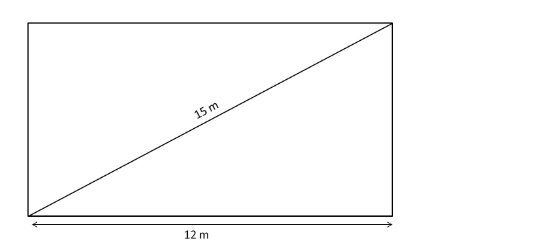# The length of a rectangular field is 12 m and the length of its diagonal is 15 m.`
Question:

The length of a rectangular field is 12 m and the length of its diagonal is 15 m. The area of the field is
(a) 108 m2
(b) 180 m2

(c) $30 \sqrt{3} \mathrm{~m}^{2}$

(d) $12 \sqrt{15} \mathrm{~m}^{2}$

Solution:

(a) 108 m2

Length of the rectangular field = 12 m
Diagonal = 15 mDiagonal $^{2}=$ Length $^{2}+$ Breadth $^{2}$

Breadth $=\sqrt{\text { Diagonal }^{2}-\text { Length }^{2}}$

$=\sqrt{15^{2}-12^{2}}$

$=\sqrt{225-144}$

$=9 \mathrm{~m}$

$\therefore$ Area of the field $=$ Length $\times$ Breadth $=12 \times 9=108 \mathrm{~m}^{2}$